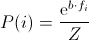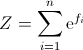io.jenetics

## Class BoltzmannSelector<G extends Gene<?,G>,N extends Number & Comparable<? super N>>

• Type Parameters:
G - the gene type.
N - the BoltzmannSelector requires a number type.
All Implemented Interfaces:
Selector<G,N>

public final class BoltzmannSelector<G extends Gene<?,G>,N extends Number & Comparable<? super N>>
extends ProbabilitySelector<G,N>

In this Selector, the probability for selection is defined as.where b controls the selection intensity, and.

fj denotes the fitness value of the jth individual.
Positive values of b increases the selection probability of the phenotype with high fitness values. Negative values of b increases the selection probability of phenotypes with low fitness values. If b is zero the selection probability of all phenotypes is set to 1/N.
Since:
1.0
Version:
4.0
Author:
Franz Wilhelmstötter

• ### Fields inherited from class io.jenetics.ProbabilitySelector

_reverter, _sorted, POPULATION_COMPARATOR
• ### Constructor Summary

Constructors
Constructor and Description
BoltzmannSelector()
Create a new BoltzmannSelector with a default beta of 4.0.
BoltzmannSelector(double b)
Create a new BoltzmannSelector with the given b value.
• ### Method Summary

All Methods
Modifier and Type Method and Description
boolean equals(Object obj)
int hashCode()
protected double[] probabilities(Seq<Phenotype<G,N>> population, int count)
Return an Probability array, which corresponds to the given Population.
String toString()
• ### Methods inherited from class io.jenetics.ProbabilitySelector

probabilities, select
• ### Methods inherited from class java.lang.Object

clone, finalize, getClass, notify, notifyAll, wait, wait, wait
• ### Constructor Detail

• #### BoltzmannSelector

public BoltzmannSelector(double b)
Create a new BoltzmannSelector with the given b value. High absolute values of b can create numerical overflows while calculating the selection probabilities.
Parameters:
b - the b value of this BoltzmannSelector
• #### BoltzmannSelector

public BoltzmannSelector()
Create a new BoltzmannSelector with a default beta of 4.0.
• ### Method Detail

• #### probabilities

protected double[] probabilities(Seq<Phenotype<G,N>> population,
int count)
Description copied from class: ProbabilitySelector

Return an Probability array, which corresponds to the given Population. The probability array and the population must have the same size. The population is not sorted. If a subclass needs a sorted population, the subclass is responsible to sort the population.

The implementer always assumes that higher fitness values are better. The base class inverts the probabilities, by reverting the returned probability array, if the GA is supposed to minimize the fitness function.
Specified by:
probabilities in class ProbabilitySelector<G extends Gene<?,G>,N extends Number & Comparable<? super N>>
Parameters:
population - The unsorted population.
count - The number of phenotypes to select. This parameter is not needed for most implementations.
Returns:
Probability array. The returned probability array must have the length population.size() and must sum to one. The returned value is checked with assert(Math.abs(math.sum(probabilities) - 1.0) < 0.0001) in the base class.
• #### hashCode

public int hashCode()
Overrides:
hashCode in class Object
• #### equals

public boolean equals(Object obj)
Overrides:
equals in class Object
• #### toString

public String toString()
Overrides:
toString in class Object

© 2007-2018 Franz Wilhelmstötter  (2018-10-28 17:23)